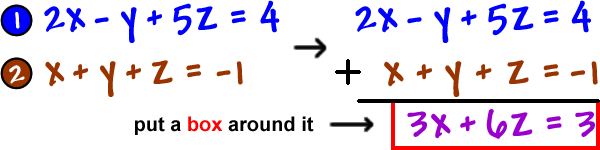OK, let's start one from scratch: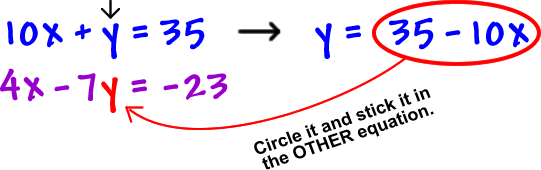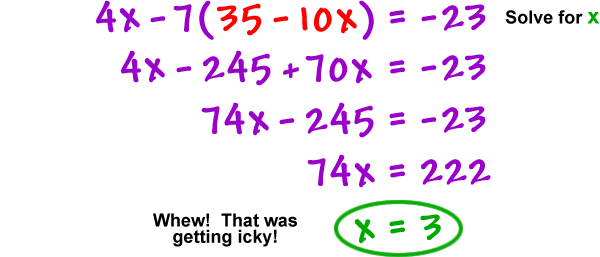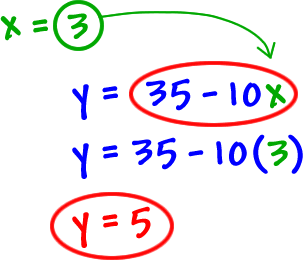The plan of attack is that we will use elimination to get rid of one variable...  we MUST use ALL three equations in this process!  One of the most common mistakes is to try to solve a 3 x 3 using only two equations...  You end up getting an answer that works in two of the equations and not in the third... which can really trick you on a test!

OK, first, let's get organized!  Label the equations so you can keep track of your work (and so can your teacher!)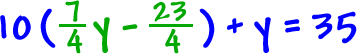For this system, the y looks like the easiest one to get rid of...  So, let's ditch the y:

 STEP 1:  Use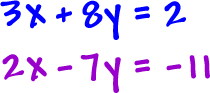and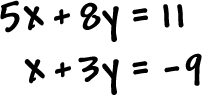to ditch the y: